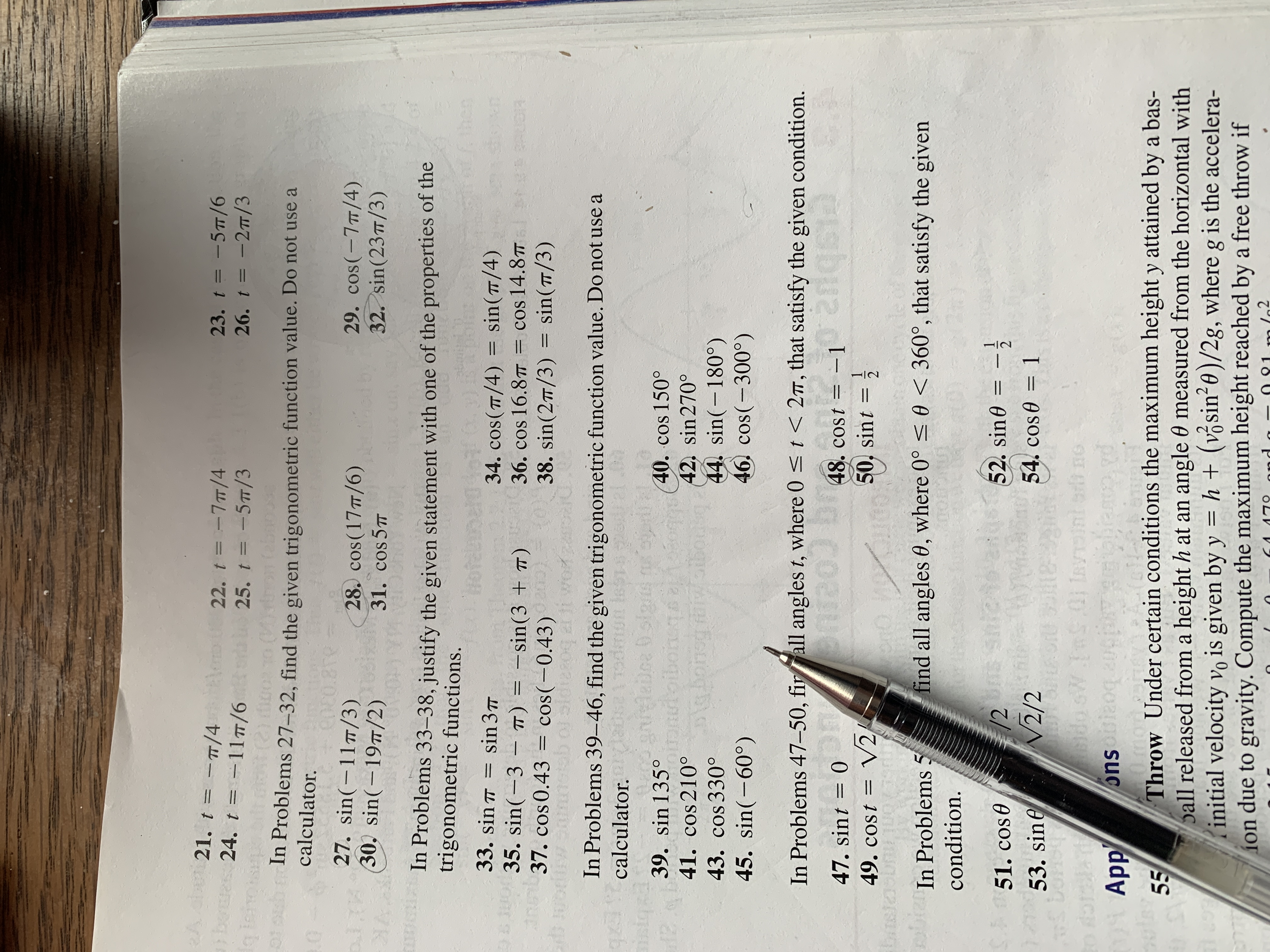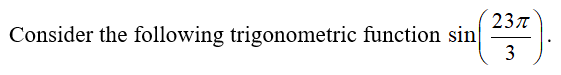# A du 21. t = -/4beues 24. t = -11#/6eo U 22. t = -7/425. t = -5T/323. t %3D -5п/626. t3D -2п/3%3DIn Problems 27-32, find the given trigonometric function value. Do not use acalculator.tionsC0E0.8Te27. sin(-117/3)28. cos(17T/6)31. cos 5729. cos(-7/4)32. sin(23/3)30, sin(-197/2)In Problems 33–38, justify the given statement with one of the properties of thetrigonometric functions.nen33. sin T =sin 3735. sin(-3 – T) = –sin(3 + )a37. cos 0.43 = cos(-0.43)34. cos(T/4) = sin(/4)= cos 14.87T38. sin(27/3) = sin(7/3)36. сos 16.87п%3D%3D%3DIn Problems 39–46, find the given trigonometric function value. Do not use acalculator.istq40. cos 150°42. sin270°44. sin(-180°)46. cos(-300°)39. sin o41. cos 210°lg bonsg faiwaibousg43. cos 330°45. sin(-60°)In Problems 47-50, firall angles t, where 0

Question
1 views

How do I do #32?help_outlineImage TranscriptioncloseA du 21. t = -/4 beues 24. t = -11#/6 eo U 22. t = -7/4 25. t = -5T/3 23. t %3D -5п/6 26. t3D -2п/3 %3D In Problems 27-32, find the given trigonometric function value. Do not use a calculator. tions C0E0.8Te 27. sin(-117/3) 28. cos(17T/6) 31. cos 57 29. cos(-7/4) 32. sin(23/3) 30, sin(-197/2) In Problems 33–38, justify the given statement with one of the properties of the trigonometric functions. nen 33. sin T = sin 37 35. sin(-3 – T) = –sin(3 + ) a37. cos 0.43 = cos(-0.43) 34. cos(T/4) = sin(/4) = cos 14.87T 38. sin(27/3) = sin(7/3) 36. сos 16.87п %3D %3D %3D In Problems 39–46, find the given trigonometric function value. Do not use a calculator. istq 40. cos 150° 42. sin270° 44. sin(-180°) 46. cos(-300°) 39. sin o 41. cos 210° lg bonsg faiwaibousg 43. cos 330° 45. sin(-60°) In Problems 47-50, firall angles t, where 0
check_circle

Step 1...

### Want to see the full answer?

See Solution

#### Want to see this answer and more?

Solutions are written by subject experts who are available 24/7. Questions are typically answered within 1 hour.*

See Solution
*Response times may vary by subject and question.
Tagged in